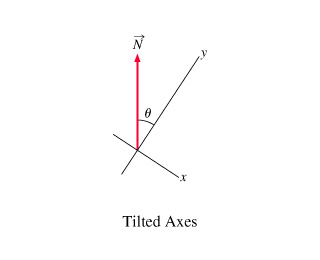# Problem: Find the components Nx and Ny of N in the tilted coordinate system. Express your answer in terms of the length of the vector N and the angle θ.

###### FREE Expert Solution

Vector N is above the negative x-axis. Thus, Nx is negative and Ny is positive.

Also, the angle is given from the y-axis.

86% (219 ratings)###### Problem Details

Find the components Nx and Ny of N in the tilted coordinate system.

Express your answer in terms of the length of the vector N and the angle θ.